# Accelerated Life Model

## Definition

Credit Scorecards based on Accelerated Life Models (Also Accelerated Failure Time Models and Duration Models)

This entry serves as the Abstract Risk Model specification of an Accelerated Life Model

## Model Context

A population of borrowers characterised by individual features (characteristics, attributes) associated with each obligor and assumed to represent credit score factors, that is, indicators of propensity to default. The population is modelled statistically for the distribution of default times.

## Model Classification

The model belongs to the following categories

• discriminative (the population characteristics are not modelled)
• parametric (a set of weights represents the parameters of the model)
• uses exclusively observed variables
• non-linear (generalized linear through exponential form)
• supervised (the historical default times represent the label)
• elementary algorithm
• frequentist approach (most commonly estimated directly on historical datasets)

## Model Description

### Response Variable

In accelerated life models the response variable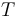$T$ follows typically an exponential or Weibull distribution. The response variable captures the timing of a Credit Event involving the i-t borrower within an observation window (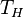$T_H$).

: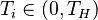$T_i \in (0, T_H)$


### Explanatory Variables

The Explanatory Variables form an n dimensional vector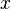$x$ that comprises potentially of both continuous and discrete (categorical) variables

### Model Parameters

A n dimensional vector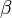$\beta$ (including the offset as the zero-th element) of parameters to be estimated

### The Functional Form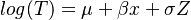$log(T) = \mu + \beta x + \sigma Z$

Regression

## Stylized Model Assumptions

• The observations of the response variable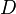$D$ are independent from each other. This assumption is manifestly not true as it is well established the defaults are correlated (Default Dependency)
• The explanatory variables combine in a linear fashion